## LetsPlayMaths.Com

WELCOME TO THE WORLD OF MATHEMATICS

# Class 7 Properties of Triangle

Types of Triangles

Scalene Triangle

Isosceles Triangle

Equilateral Triangle

Acute Triangle

Right-Angled Triangle

Obtuse Triangle

Perimeter of a Triangle

Properties of Triangle

Exterior Angles of a Triangle

Sum of Lengths of Two Sides of a Triangle

Pythagoras Theorem

Properties of Triangle Test

Properties of Triangle Worksheet

## Types of Triangles

Triangles are named on the basis of the lengths of their sides and the measures of their angles. Different types of triangles are given below.

1. Scalene Triangle
2. Isosceles Triangle
3. Equilateral Triangle
4. Acute Triangle
5. Right Triangle
6. Obtuse Triangle

## Scalene Triangle

A triangle whose all three sides length are not equal is known as scalene triangle. Figure below shows an example of scalene triangle.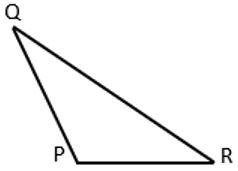PQ ≠ PR ≠ QR

## Isosceles Triangle

A triangle whose two sides are equal is known as an isosceles triangle. Figure below shows an example of isosceles triangle.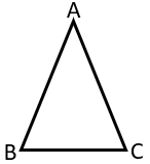Here, AB = AC

## Equilateral Triangle

A triangle whose all sides are equal to one another is known as equilateral triangle. Figure below shows an example of equilateral triangle.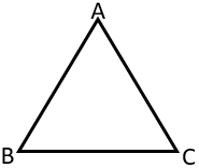Here, AB = BC = AC and ∠ABC = ∠BAC = ∠ACB = 60°

## Acute Triangle

A triangle whose all the angles are acute is known as acute angled triangle or acute triangle.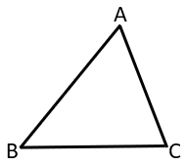Equilateral triangle is also an acute angled triangle.

## Right-Angled Triangle

A triangle whose one angle is a right angle is known as a right-angled triangle.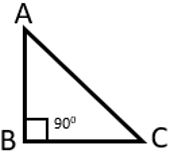Triangle ABC is a right-angled triangle, where ∠ABC = 90°

## Obtuse Triangle

A triangle whose one angle is more than 90° is known as obtuse-angled triangle.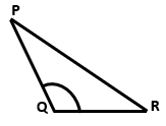Here, triangle PQR is an obtuse angled triangle, where ∠PQR is an obtuse angle.

## Perimeter of a Triangle

The sum of the lengths of the sides of a triangle is called its perimeter. Let's assume a, b and c are the three sides of a triangle, then its perimeter = a + b + c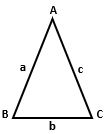## Properties of Triangle

1. The sum of the angles of a triangle is 180°
2. A triangle can not have more than one right angle
3. A triangle can not have more than one obtuse angle
4. In a right-angled triangle, the other two angles are acute, and their sum is 90°

Let's see some examples to understand these properties.

Example 1. If two angles of a triangle are 65° and 45°, then find the third angle.

Solution. As we know sum of all the angles of a triangle is 180°. Two angles are provided, they are 65° and 45°. Let's assume the third angle is 'x'.

x + 65° + 45° = 180°

⇒ x = 180° − 110°

⇒ x = 70°

Hence, the third angle is 70°.

Example 2. Find all the angles of right-angled isosceles triangle.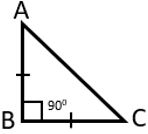Solution. Here, ∠ABC = 90° and AB = BC

∠BAC = ∠BCA = x°

As we know ∠ABC + ∠BAC + ∠BCA = 180°

⇒ 90° + x° + x° = 180°

⇒ 90° + 2x° = 180°

⇒ 2x° = 180° − 90°

⇒ 2x° = 90°

⇒ x° = 45°

So, ∠ABC = 90°, ∠BAC = 45° and ∠BCA = 45°

Example 3. If the angles of a triangle are in the ratio 2 : 3 : 4, then find the three angles of the triangle.

Solution. Let's assume all the three angles are 2x°, 3x°, and 4x°.

As we know sum of all the angles are equal to 180°

2x° + 3x° + 4x° = 180°

⇒ 9x° = 180°

⇒ x° = 180°9

⇒ x° = 20°

So, the angles of the triangles are 40°, 60° and 80°.

Example 4. The sum of two angles of an isosceles triangle is equal to it's third angle. Determine the measures of all the angles.

Solution. Let's assume PQR is the triangle and ∠P, ∠Q, and ∠R it's angles.

∠P + ∠Q = ∠R

As we know ∠P + ∠Q + ∠R = 180°

⇒ ∠R + ∠R = 180°

⇒ 2∠R = 180°

⇒ ∠R = 90°

As it is a right-angled isosceles triangle, it's ∠P = ∠Q

So, ∠P = ∠Q = 45° and ∠R = 90°

Example 5. One of the acute angles of a right-angled triangle is 50°. Find the other acute angle.

Solution. Let's assume the other acute angle is p°.

As we know, sum of all the angles of a triangle is 180°.

90° + 50° + p° = 180°

⇒ p° = 180° − 140°

⇒ p° = 40°

So, the third acute angle is 40°.

Example 6. In below given figure DE || BC, ∠A = 30°, and ∠C = 40°. Find the value of x, y and z.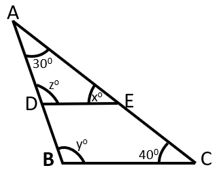Solution. In triangle ABC, we have ∠A = 30°, and ∠C = 40°

∠A + ∠B + ∠C = 180°

⇒ 30° + y° + 40° = 180°

⇒ y° + 70° = 180°

⇒ y° = 110°

Here, DE || BC and transversal AB intersect them at point B and D. So corresponding angles will be equal.

⇒ y° = z°

⇒ z° = 110°

Similarly, transversal AC intersect them at point E and C. So corresponding angles will be equal.

∠AED = ∠C

⇒ x° = 40°

Hence x° = 40°, y° = 110° and z° = 110°

## Exterior Angles of a Triangle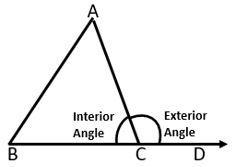In the above figure ABC is a triangle and its side BC is extended to D. Here, ∠ACD is known as exterior angle and ∠ACB is known as interior angle. Here, ∠ACD and ∠ACB are adjacent supplementary angles. Interior Angle + Exterior Angle = 180° ∠BAC and ∠ABC is known as interior opposite angles of ∠ACD ∠BAC + ∠ABC = ∠ACD Let's see some examples to understand it better.

Example 1. Find the value of 'x' in the below given figure.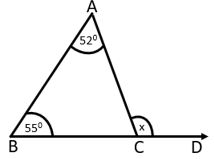Solution. As we know, exterior angle of a triangle is equal to its two interior opposite angles.

x = 55° + 52°

⇒ x = 107°

Example 2. Find the measures of ∠ABC and ∠BAC in the below given figure.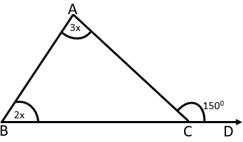Solution. Exterior angle of a triangle is equal to its two interior opposite angles.

2x + 3x = 150°

⇒ 5x = 150°

⇒ x = 150°5

⇒ x = 30°

Hence, ∠ABC = 60° and ∠BAC = 90°

Example 3. Find the value of 'p' and 'q' in the below given figure.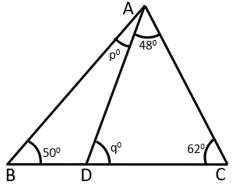Solution. From the above figure we can see two triangles ABC and ADC.

Let's consider triangle ADC first.

q° + 48° + 62° = 180°

⇒ q° = 180° − 110°

⇒ q° = 70°

Now consider, triangle ABC.

∠ABC + ∠BAC + ∠ACB = 180°

⇒ 50° + p° + 48° + 62° = 180°

⇒ p° + 160° = 180°

⇒ p° = 180° − 160°

⇒ p° = 20°

Hence, p° = 20° and q° = 70°.

Example 4. Find the value of 'x', 'y', and 'z' in the below given figure.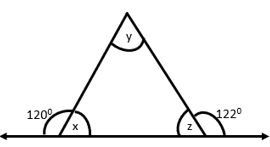Solution. Let's find out angle 'x' and 'z' using linear pair rule.

x + 120° = 180°

⇒ x = 60°

z + 122° = 180°

⇒ z = 58°

x + y + z = 180°

⇒ 60° + y + 58° = 180°

⇒ y = 180° − 60° − 58°

⇒ y = 62°

Hence, x = 60°, y = 62° and z = 58°

## Sum of Lengths of Two Sides of a Triangle

Sum of length of any two sides of a triangle is greater than the length of its third side. This rule is applicable for any kind of triangle.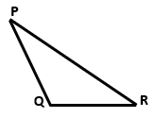Here, PQ + QR > PR

PQ + PR > QR

PR + QR > PQ

Let's see some examples to understand it better.

Example 1. Is it possible to have a triangle with the following sides?

3 cm, 5 cm, 7cm

Solution. 3 cm + 5 cm > 7 cm

3 cm + 7 cm > 5 cm

5 cm + 7 cm > 3 cm

Hence, it is proved that sum of lengths of any two sides is greater than the length of third side. Therefore, the triangle is possible.

Example 2. Is it possible to have a triangle with the following sides?

10 cm, 4 cm, 5 cm

Solution. 5 cm + 4 cm < 10 cm

Here, sum of lengths of any two sides is less than the third sides. So triangle formation can not be possible.

## Pythagoras Theorem

In a right-angled triangle, the square of the hypotenuse equals to the sum of the squares of its remaining two sides.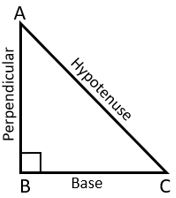(Hypotenuse)2 = (Base)2 + (Perpendicular)2

Let's assume, Hypotenuse = c

Base = a

Perpendicular = b

c2 = a2 + b2

Example 1. The hypotenuse of a right-angled triangle is 15 cm long and length of the base is 5 cm. Find the height of the triangle.

Solution. Let's assume ABC is a right-angled triangle as shown in the below figure.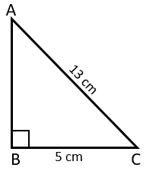Here, AC = 13 cm and BC = 5 cm

According to Pythagoras theorem AB2 + BC2 = AC2

⇒ AB2 + 52 = 132

⇒ AB2 = 132 − 52

⇒ AB2 = 169 − 25

⇒ AB2 = 144

⇒ AB =  144

⇒ AB = 12 cm

Hence, height of the triangle is 12 cm.

Example 2. Find the value of 'x' in the below given figure.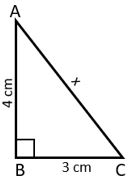Solution. As we know, AB2 + BC2 = AC2

AC2 = 42 + 32

⇒ AC2 = 16 + 9

⇒ AC =  25

⇒ AC = 5 cm

Hence, x is equal to 5 cm.

Example 3. A 25 m long ladder riches a roof of 20 m high from the ground on placing it against the wall. How far is the foot of the ladder from the wall?

Solution. Let's assume AB is the ladder, B is the roof and CB is the height of the wall and AC is the distance between wall and ladder.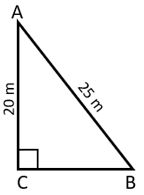AC2 + BC2 = AB2

⇒ BC2 = AB2 − AC2

⇒ BC2 = 252 − 202

⇒ BC2 = 625 − 400

⇒ BC =  225

⇒ BC = 15 m

Hence, the ladder is 15 m away from the wall.

## Properties of Triangle Test

Properties of Triangle Test - 1

Properties of Triangle Test - 2

Properties of Triangle Test - 3

## Class-7 Properties of Triangle Worksheet

Properties of Triangle Worksheet - 1

Properties of Triangle Worksheet - 2

Properties of Triangle Worksheet - 3